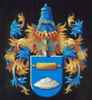# Report: Dagen verschil tussen dood en leven

Description: / Individuals: frequency distribution of difference (in "absolute" weeks) between day/month of birth and day/month of death

Matches 1 to 27 of 27   » Comma-delimited CSV file

 # Diffence_in_weeks Amount Graphik 1 0 16 === 2 1 14 === 3 2 12 == 4 3 9 == 5 4 8 == 6 5 8 == 7 6 13 === 8 7 8 == 9 8 6 = 10 9 5 = 11 10 14 === 12 11 10 == 13 12 4 = 14 13 9 == 15 14 13 === 16 15 10 == 17 16 9 == 18 17 13 === 19 18 5 = 20 19 11 == 21 20 13 === 22 21 14 === 23 22 7 = 24 23 8 == 25 24 13 === 26 25 11 == 27 26 12 ==